# Bioenergetics MCQ

## 21 Questions MCQ Test Mock Test Series of IIT JAM Biotechnology | Bioenergetics MCQ

Description
Attempt Bioenergetics MCQ | 21 questions in 50 minutes | Mock test for IIT JAM preparation | Free important questions MCQ to study Mock Test Series of IIT JAM Biotechnology for IIT JAM Exam | Download free PDF with solutions
QUESTION: 1

### In which of the following conditions the Gibbs free energy change shall be negative when the

Solution:

Negative Gibbs free energy change for a process indicates that the process is spontaneous

QUESTION: 2

### Gibbs free energy change does not depend on which of the following factors?

Solution:

The value of Gibbs tree energy change can be calculated using the formula or equation ΔG = ΔH - TΔS. This relationship indicates that it is not dependent on number of moles of the reacting species.

QUESTION: 3

### Which of the following information is not derived from first law of thermodynamics

Solution:

Randomness of the universe is always increasing.  This is stated in second law of thermodynamics

QUESTION: 4

If the value of ΔH and ΔS are positive, which of the following statements will be true.

Solution:

Option (a) is correct as according to Gibb's free energy equation ΔG = ΔH - TΔS.
Now if. ΔH = —ve (as given in option (a)) (at low temperature) and ΔS = +ve.
then ΔG = -ve (spontaneous)
option (b) is correct if
ΔH = +ve (as given in option (b)) and ΔS = +ve. Also if ΔS > ΔH (conditional), then ΔG = -ve (spontaneous)
Option (c) is correct
If ΔS = —ve (as given in option (c)) and ΔH = -ve. Also ΔH > ΔS (conditional) then. ΔG = -ve (spontaneous)

QUESTION: 5

Which of the following complex of electron transport chain is not involved in proton pumping  across the inner mitochondrial membrane.

Solution:

Complex I  (NADH dehydrogenase), complex III (cytochrome bc 1 complex), and complex IV (cytochrome c oxidase) of electron transport chain (ETC) pump protons across the inner mitochondrial membrane during electron transport. However, complex II (succinate dehydrogenase) which receives electrons from FADH2 is not involved in proton pumping during electron transport.

QUESTION: 6

Which of the following options consist of combination of inhibitors which inhibit the same target.

Solution: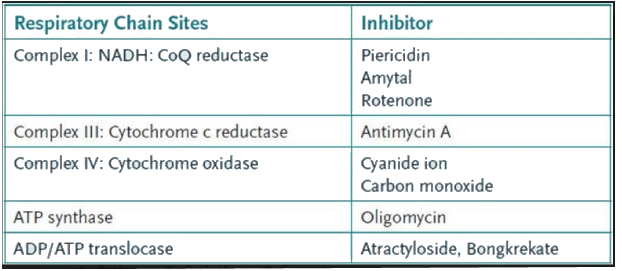QUESTION: 7

In which of the following conditions the Gibbs free energy change can be negative

Solution:

Option (a) is correct as according to Gibb's free energy equation ΔG = ΔH - TΔS.
Now if. ΔH = -ve (as given in option (a)) (at low temperature) and ΔS = +ve. then ΔG = -ve (spontaneous)
ption (b) is correct if
ΔH = +ve (as given in option (b)) and ΔS = +ve. Also if ΔS > ΔH (conditional), then ΔG = -ve (spontaneous)
Option (c) is conect
If ΔS = -ve (as given in option (c)) and ΔH = -ve. Also ΔH > ΔS (conditional) then. ΔG = -ve (spontaneous)

QUESTION: 8

What is true about the Gibbs free energy change at equilibrium?

Solution:

The value of Gibbs free energy change (ΔG) is zero at equilibrium as the direction of reaction is neither forward nor backward at equilibrium. However, the value of standard Gibbs fr ee energy change (ΔG°) cannot be zero at equilibrium unless the value of equilibrium constant is one (that will be a special case). However at equilibrium ΔG° is minimum
(We define ΔG° (pronounced “delta G naught prime*’) as the fr ee energy change of a reaction under “standard conditions" which are defined as:

• All reactants and products are at an initial concentration of 1.0M
• Pressure of 1.0 atm
• Temperature is 25°C
QUESTION: 9

As one molecule of NADH is oxidized via the respiratory chain:

Solution:

Oxidation of one molecule of NADH via the respiratory chain generates 2.5 molecules of ATP in total. One is formed via complex I, 1 via complex III and 0.5 via complex IV. Complex II is not involved in proton pumping.

QUESTION: 10

Arrange the following electron carries of electron transport chain in ascending order of redox potential. FMN, cytochrome c, cytochrome a3 and O2

Solution:

In the below figure, electron carriers of electron transport chain (ETC) are arranged in increasing order of their redox potential. Oxygen has the most positive redox potential and is the terminal electron acceptor in ETC.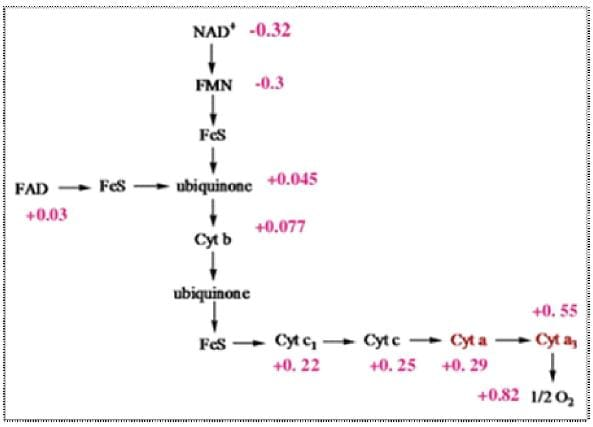QUESTION: 11

What is the mechanism of action of oligomycin

Solution:

Oligomycin is a inhibitor of ATP synthase. Oligomycin inhibits ATP synthase by blocking its proton channel (Fo subunit) which is necessary for oxidative phosphorylation of ADP to ATP.

QUESTION: 12

Which of the following molecules produces highest energy on breakdown of its high energy bond

Solution:

ATP produces high amount of energy, yet it is not the highest free energy molecule. The free energy of ATP hydrolysis is – 30 kJ per mole, while PEP has much higher energy of breakdown i.e. ~ 54 kJ per mole.

*Multiple options can be correct
QUESTION: 13

Which of the following is not true for energy currency of the cell

Solution:

PEP has the highest Gibb’s energy of hydrolysis and ATP has two high energy bonds.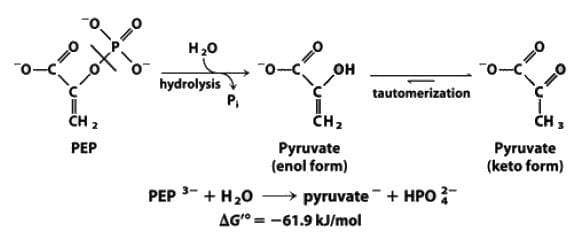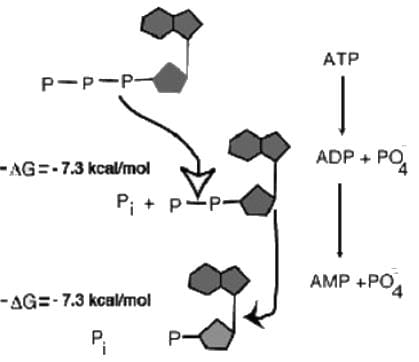*Multiple options can be correct
QUESTION: 14

Which of the following is true about the sign convention of work done and heat

Solution: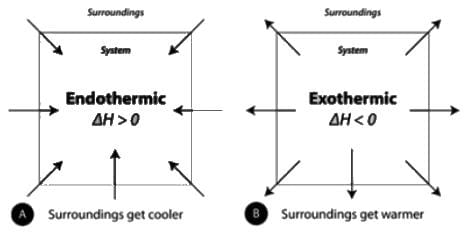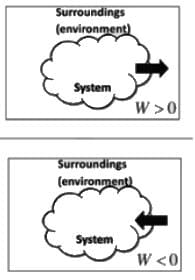*Multiple options can be correct
QUESTION: 15

Which one of the following statements about cytochromes P450 is/are CORRECT?

Solution:

Option (b) is incorrect as cytochromes p450 are located mainly in the endoplasmic reticulum but they are also found in mitochondria in some tissues. Cytochrome P450 enzymes also function to metabolize potentially toxic compounds, including drugs and products of endogenous metabolism such as bilirubin, principally in the liver. The Human Genome Project has identified 57 human genes coding for the various cytochrome P450 enzymes.

*Multiple options can be correct
QUESTION: 16

The chemiosmotic theory of Peter Mitchell proposes a mechanism for the tight coupling of electron transport via the respiratory chain to the process of oxidative phosphorylation.Which of the following options is predicted by the theory?

Solution:

Option (b) is incorrect as the electrochemical potential difference across the inner mitochondrial membrane caused by electron transport must be negative on the matrix side so that protons are forced to reenter via the ATP synthase to discharge the gradient and promote ATP synthesis.

*Multiple options can be correct
QUESTION: 17

Which of the following statements is false

Solution:

Statement (a) is false
Complex III is involved in proton pumping. Complex II is not involved in proton pumping
Statement (b) is false
DNP and FCCP are uncouplers. They uncouple electron transport chain from oxidative phosphorylation. Atractyloside is not a uncoupler. Atractyloside is a ADP/ATP translocase inhibitor.
Statement (c) is false
Oxygen is the terminal electron acceptor in ETC (electron transport chain)
Statement (d) is false
Reducing power of NADH is more than FADH2. 1 molecule of 1 NADH gives 2.5 ATP and 1 molecule of FADH2 gives 1.5 ATP.

*Answer can only contain numeric values
QUESTION: 18

The value of standard reduction potential is –30 mV for an oxidation reaction involving 2 electrons. The standard  Gibbs free energy (in kilojoule) for this reaction is _________ (F = 96500 Joule/mol). (Answer upto one decimal place)

Solution:

This question can be solved using the formula ΔG° = —nFE°
n is equal to the number of electrons involved and potential must be converted to volts in order to obtain answer in joules.
i.e ΔGº= —2 x 96500 x (-0.03) = 5790 Joules = 5.79 kJ = 5.8 kJ.

*Answer can only contain numeric values
QUESTION: 19

The Gibbs free  energy change for conversion of compound X to Compound Y is 20 kJ mol–1 and it is a non spontaneous reaction.
Also hydrolysis of ATP is a coupled reaction and provides –30 kJ/mol energy on hydrolysis.
From the information given above, the Gibbs free energy change for the reaction
Compound X + ATP —> Compound Y + ADP is__________ (in kJ mol-1) (answer in integer)

Solution:

X→Y ; ΔG = 20 kJ mol-1 ... (1)
ATP →ADP + pi; ΔG = —30 kJ mot-1 ... (2)
X + ATP → Y + ADP + p1  ΔG = 20 — 30 = —10 kJ mol-1
In order to calculate the net Gibbs energy of a reaction, if the Gibbs energy of two separate reactions are given, add or substract the two given reactions in such a way that you obtain the desired reaction. In the same way energies are also added or subtracted.

*Answer can only contain numeric values
QUESTION: 20

The DG' for the complete oxidation of lactic acid to CO2 and H2O calculated from the information given below is ______ (in cal/mole).
Moles of ATP that could be synthesized in the above process at 40% efficiency is _________ (1 mole of ATP = 7300 calories) (answer in integer)
1. glucose → 2 lactic acid                       ΔG' = —52.000cal/mole
2. glucose + 6O2 → 6 CO2 + 6H20        ΔG' = —686,000 cal/mole

Solution:

We want to calculate the ΔG' of following reaction
Lactic acid +3 02,→ 3 CO2 + 3 H20 .....(1)
glucose → 2 lactic acid ΔG,' = -52.000 callmok (Given) ....(3)
2Lactic acid +6 024 6 CO2 + 6 H2O ΔG2' =? Cal/mole ... (4)
(equation (4) is obtained by multiplying equation (1) by 2)
Add equation 3 and 4. we get
Glucose + 6O→ 6 CO2 + 6 H2O            ΔG3' = -686,000 cal/mole (Given)
ΔG3'= +ΔG2'
–686,000 cal/mole        = –52,000 cal/mole + ΔG2'
ΔG2'= -634,000 cal/mole
Oxidation of 2 moles of lactic acid gives -634.000 cal/mole. so 1 mole gives -317.000 cal/moles (b) At 40% efficiency = 0.40 x 317000 cal/mole = 127000 cal/mol (Approx)
1ATP gives 7300 cal
No. of ATP required = 127000/7300 = 17.4 ATP

*Answer can only contain numeric values
QUESTION: 21

If the ratio of ATP: ADP is 105 :1, the Gibbs free energy change of hydrolysis of ATP at 37°C will be _____ (kilo Joule). Given that standard Gibbs free energy change is –30 kJ/ mol. (Answer in integer)

Solution:

This question can be solved using the formula ΔG =ΔGº + 2.303 RT log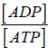ΔG = -30.000 + 2.303 x 8.31 x 310 x log 10-5
ΔG = -30.000 + 5933 x logl0-5
ΔG = -30000 + 5933 x (-5)
ΔG = -30000 - 29665
ΔG = -59665 = - 59.665 kJ = - 60 kJUse Code STAYHOME200 and get INR 200 additional OFF Use Coupon Code